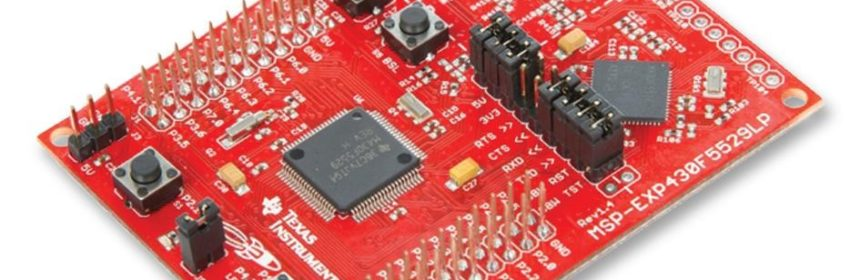## Tinkering TI MSP430F5529ADC interrupt is as important as timer and communication interrupts. We can do other tasks while ADC is performing a conversion.

### Code Example

```#include "driverlib.h"
#include "delay.h"
#include "lcd.h"
#include "lcd_print.h"

unsigned long cnt = 0;

void clock_init(void);
void GPIO_init(void);

{
{
case  0: break;   //Vector  0:  No interrupt
case  2: break;   //Vector  2:  ADC overflow
case  4: break;   //Vector  4:  ADC timing overflow
{
break;
}
case  8: break;   //Vector  8:  ADC12IFG1
case 10: break;   //Vector 10:  ADC12IFG2
case 12: break;   //Vector 12:  ADC12IFG3
case 14: break;   //Vector 14:  ADC12IFG4
case 16: break;   //Vector 16:  ADC12IFG5
case 18: break;   //Vector 18:  ADC12IFG6
case 20: break;   //Vector 20:  ADC12IFG7
case 22: break;   //Vector 22:  ADC12IFG8
case 24: break;   //Vector 24:  ADC12IFG9
case 26: break;   //Vector 26:  ADC12IFG10
case 28: break;   //Vector 28:  ADC12IFG11
case 30: break;   //Vector 30:  ADC12IFG12
case 32: break;   //Vector 32:  ADC12IFG13
case 34: break;   //Vector 34:  ADC12IFG14
default: break;
}
}

void main(void)
{
unsigned long volts = 0;

WDT_A_hold(WDT_A_BASE);

clock_init();
GPIO_init();

LCD_init();
LCD_clear_home();

LCD_goto(0, 0);

LCD_goto(0, 1);
LCD_putstr("Volts/mV :");

while(1)
{
volts = ((cnt * 3300) / 4095);
print_I(11, 0, cnt);
print_I(11, 1, volts);
delay_ms(100);
};
}

void clock_init(void)
{
PMM_setVCore(PMM_CORE_LEVEL_3);

GPIO_setAsPeripheralModuleFunctionInputPin(GPIO_PORT_P5,
(GPIO_PIN4 | GPIO_PIN2));

GPIO_setAsPeripheralModuleFunctionOutputPin(GPIO_PORT_P5,
(GPIO_PIN5 | GPIO_PIN3));

UCS_setExternalClockSource(XT1_FREQ,
XT2_FREQ);

UCS_turnOnXT2(UCS_XT2_DRIVE_4MHZ_8MHZ);

UCS_turnOnLFXT1(UCS_XT1_DRIVE_0,
UCS_XCAP_3);

UCS_initClockSignal(UCS_FLLREF,
UCS_XT2CLK_SELECT,
UCS_CLOCK_DIVIDER_4);

UCS_initFLLSettle(MCLK_KHZ,
MCLK_FLLREF_RATIO);

UCS_initClockSignal(UCS_SMCLK,
UCS_XT2CLK_SELECT,
UCS_CLOCK_DIVIDER_2);

UCS_initClockSignal(UCS_ACLK,
UCS_XT1CLK_SELECT,
UCS_CLOCK_DIVIDER_1);
}

void GPIO_init(void)
{
GPIO_setAsOutputPin(GPIO_PORT_P4,
GPIO_PIN7);

GPIO_setAsPeripheralModuleFunctionInputPin(GPIO_PORT_P6,
GPIO_PIN0);
}

{

&configureMemoryParam);

__enable_interrupt();

}```

### Explanation

This example is similar to the last one in many aspects. I won’t be going through all settings. I’ll only highlight the key differences.

This time an external ADC channel is used and so we have to initialize the alternative role of its pin.

`GPIO_setAsPeripheralModuleFunctionInputPin(GPIO_PORT_P6, GPIO_PIN0);`

In the ADC12 initialization, the external input pin or the ADC channel’s physical pin is set after its GPIO initialization. Unlike the last ADC example in which we used internal 1.5V reference source, the positive reference source for ADC this time is set to AVCC. AVSS is the negative voltage source for both examples. AVCC is same as the system bus voltage, i.e. 3.3V.

```configureMemoryParam.inputSourceSelect = ADC12_A_INPUT_A0;

Since we are using interrupt method, relevant interrupt flag is cleared first and then enabled.

```ADC12_A_clearInterrupt(ADC12_A_BASE, ADC12IFG0);
__enable_interrupt();```

In this case the 0th ADC interrupt flag is used since the channel to be read is the 0th channel of the ADC. Don’t confuse interrupt vector number with interrupt flag number. Interrupt vector number for 0th ADC interrupt flag is 6.

```#pragma vector = ADC12_VECTOR
{
{
case  0: break;   //Vector  0:  No interrupt
case  2: break;   //Vector  2:  ADC overflow
case  4: break;   //Vector  4:  ADC timing overflow
{
break;
}
case  8: break;   //Vector  8:  ADC12IFG1
case 10: break;   //Vector 10:  ADC12IFG2
case 12: break;   //Vector 12:  ADC12IFG3
case 14: break;   //Vector 14:  ADC12IFG4
case 16: break;   //Vector 16:  ADC12IFG5
case 18: break;   //Vector 18:  ADC12IFG6
case 20: break;   //Vector 20:  ADC12IFG7
case 22: break;   //Vector 22:  ADC12IFG8
case 24: break;   //Vector 24:  ADC12IFG9
case 26: break;   //Vector 26:  ADC12IFG10
case 28: break;   //Vector 28:  ADC12IFG11
case 30: break;   //Vector 30:  ADC12IFG12
case 32: break;   //Vector 32:  ADC12IFG13
case 34: break;   //Vector 34:  ADC12IFG14
default: break;
}
}```

ADC interrupt triggers when a new result is loaded in ADC memory location. Inside the interrupt ADC memory location 0 is read to get the conversion result, i.e. ADC count. When the ADC memory is accessed, its interrupt flag is automatically cleared.

In the main loop, the ADC count derived from the ADC interrupt is converted to voltage. Both the voltage and ADC count are displayed on an alphanumerical LCD.

```volts = ((cnt * 3300) / 4095);
print_I(11, 0, cnt);
print_I(11, 1, volts);
delay_ms(100);```

### Related Posts

• Surya k sHello
I am doing project of msp430g2553 interface(using i2c communication) with temp 100(temperature sensor) and try to read the temperature in dispaly(16*2) but didn’t get the out put (using code composer studio) can u share me any example code for this project

Thank you sir,

•Which sensor? Did you use pullup resistors for SDA-SCL pins?

• CristianWhere is lcd_print.h?

•You want the truth? TI makes and sell “underpowered micros”, you know? Low everything, not only the power but also peripherals. So the price is not justified.

Otherwise, if I’ll move there, I’ll introduce them to my small hobby projects – there are still some advantages.

•I may even make a visual configuration tool of my own for them…

•Yeah the prices of TI products are higher than other manufacturers but I don’t think the hardware peripherals are inferior.

•Not inferior but in not enough numbers compared to STM32.

•True